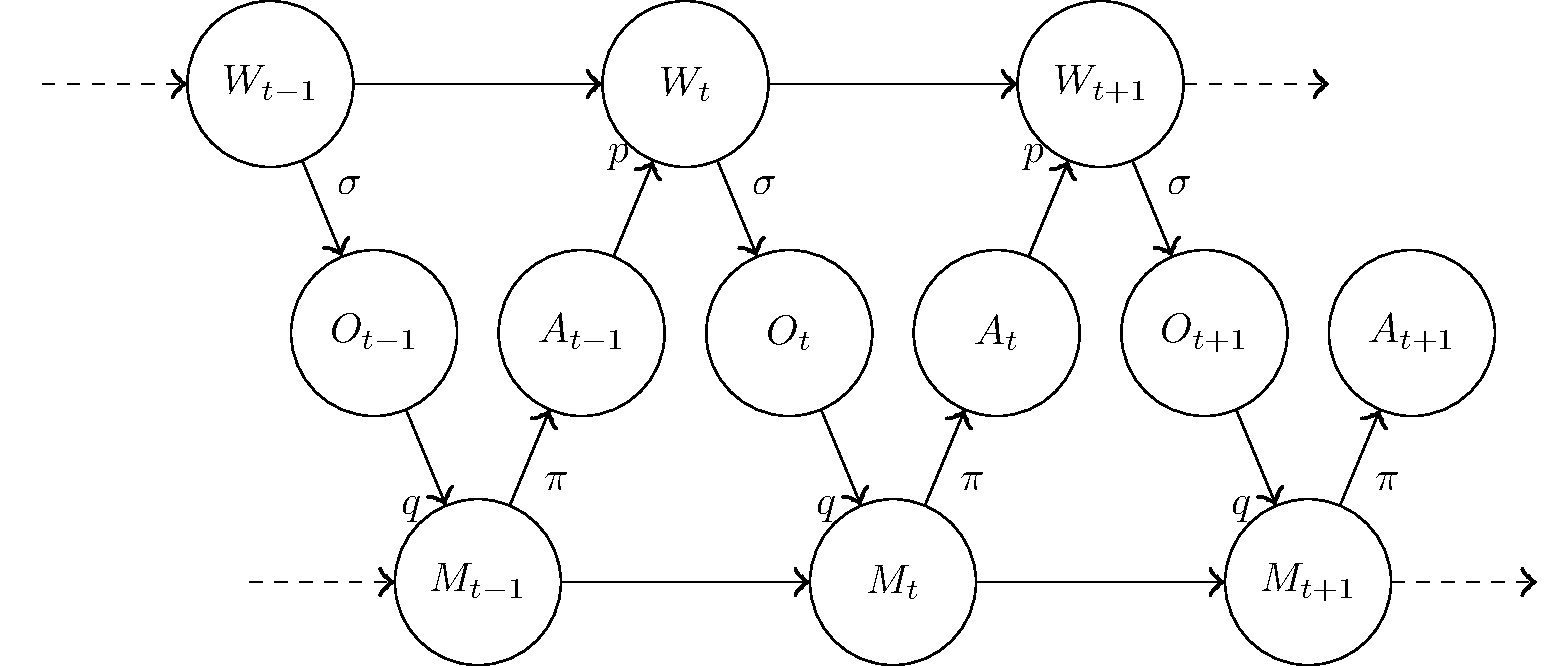# 10

Personal Blog

A reinforcement-learning agent is interacting with its environment through the perception of observations and the performance of actions.

We describe the influence of the world on the agent in two steps. The first is the generation of a sensory input Ot based on the state of the world Wt. We assume that this step is in accordance with the laws of physics, and out of anyone's hands. The second step is the actual changing the agent's mind to a new state Mt. The probability distributions of these steps are, respectively, σ(Ot|Wt) and q(Mt|Mt-1,Ot).

Similarly, the agent affects the world by deciding on an action At and performing it. The designer of the agent can choose the probability distribution of actions π(At|Mt), but not the natural laws p(Wt+1|Wt,At) saying how these actions change the world.So how do we choose q and π? Let's first assume that it matters how. That is, let's assume that we have some preference over the results of the process, the actual values that all the variables take. Let's also make some further assumptions regarding this preference relation:

1. The first assumption will be the standard von Neumann-Morgenstern rationality. This is a good opportunity to point out a common misconception in the interpretation of that result. It is often pointed out that humans, for instance, are not rational in the VNM sense. That is completely beside the point.

The agent doesn't choose the transitions q and π. The agent is these transitions. So it's not the agent that needs to be rational about the preference, and indeed it may appear not to be. If the agent has evolved, we may argue that evolutionary fitness is VNM-rational (even if the local-optimization process leads to sub-optimal fitness). If humans design a Mars rover to perform certain functions, we may argue that the goal dictates a VNM-rational preference (even if we, imperfect designers that we are, can only approximate it).

2. The second assumption will be that the preference is strictly about the territory Wt, never about the map Mt. This means that we are never interested in merely putting the memory of the agent in a "good" state. We need it to be followed up by "good" actions.

You may think that an agent that generates accurate predictions of the stock market could be very useful. But if the agent doesn't follow up with actually investing well, or at least reliably reporting its findings to someone who does, then what good is it?

So we are going to define the preference with respect to only the states W1, ..., Wn and the actions A1, ..., An. If the observations are somehow important, we can always define them as being part of the world. If the agent's memory is somehow important, it will have to reliably communicate it out through actions.

3. The third assumption will be the sunk cost assumption. We can fix some values of the first t steps of the process, and consider the preference with respect to the remaining n-t steps. This is like finding ourselves in time t, with a given t-step history, considering what to do next (though of course we plan for that contingency ahead of time). Our assumption is that we find that the preference is the same, regardless of the fixed history.

This last assumption gives rise to a special version of the von Neumann-Morgenstern utility theorem. We find that what we really prefer is to have as high as possible the expectation of a utility function with a special structure: the utility ut depends only on Wt, At and the expectation of ut+1 from the following step:

ut(Wt,At,E(ut+1))

This kind of recursion which goes backwards in time is a recurring theme in reinforcement learning.

We would like to have even more structure in our utility function, but this is where things become less principled and more open to personal taste among researchers. We base our own taste on a strong intuition that the following could, one day, be made to rely on much better principles than it currently does.

We will assume that ut is simply the summation of some immediate utility and the expectation of future utility:

ut(Wt,At,E(ut+1)) = R(Wt,At) + E(ut+1)

Here R is the Reward, the additional utility that the agent gets from taking the action At when the world is in state Wt. It's not hard to see that we can write down our utility in closed form, as the total of all rewards we get throughout the process

R(W1,A1) + R(W2,A2) + ... + R(Wn,An)

As a final note, if the agent is intended to achieve a high expectation of the total reward, then it may be helpful for the agent to actually observe its reward when it gets it. And indeed, many reinforcement learning algorithms require that the reward signal is visible to the agent as part of its observation each step. This can help the agent adapt its behavior to changes in the environment. After all, reinforcement learning means, quite literally, adaptation in response to a reward signal.

However, reinforcement learning can also happen when the reward signal is not explicit in the agent's observations. To the degree that the observations carry information about the state of the world, and that the reward is determined by that state, information about the reward is anyway implicit in the observations.

In this sense, the reward is just a score for the quality of the agent's design. If we take it into account when we design the agent, we end up choosing q and π so that the agent will exploit the implicit information in its observations to get a good reward.